# Problems in Trees

## PROBLEM WRITE A NON RECURSIVE VERSION OF PREORDER

Program

```
/**************** stack.c **********************/
#include

#define SUCCESS 0
#define ERROR -1

struct tnode;

typedef struct tnode *stype;
typedef struct snode snode;

struct snode {
stype data;
snode *next;
};

snode *stop = NULL; // signifies empty stack.

int sPush( stype data ) {
/*
* push data at stop.
*/
snode *ptr = (snode *)malloc( sizeof(snode) );
ptr->data = data;
ptr->next = stop;
stop = ptr;

return SUCCESS;
}

stype sPop() {
/*
* returns data at stop.
*/
stype data;
snode *ptr;

if( sEmpty() ) {
fprintf( stderr, "ERROR : popping from empty stack." );
return (stype)NULL;
}
data = stop->data;
ptr = stop;
stop = stop->next;
free(ptr);

return data;
}

int sEmpty() {
return ( stop == NULL );
}

/**************** queue.c **********************/
#include

#define SUCCESS 0
#define ERROR -1

struct tnode;

typedef struct tnode *qtype;
typedef struct qnode qnode;

struct qnode {
qtype data;
qnode *next;
};

qnode *front = NULL, // signifies empty queue.
*rear = NULL;

int qInsert( qtype data ) {
/*
* inserts data at rear.
*/
qnode *ptr = (qnode *)malloc( sizeof(qnode) );
ptr->data = data;
ptr->next = NULL;
if( qEmpty() )
front = ptr;
else
rear->next = ptr;
rear = ptr;

return SUCCESS;
}

qtype qRetrieve() {
/*
* retrieve data from front and remove it from queue.
*/
qtype data:
if( qEmpty() ) {
fprintf( stderr, "ERROR : retrieving from empty queue." );
return (qtype)NULL;
}
data = front->data;
front = front->next;
if( qEmpty() ) // last node removed.
rear = NULL;
return data;
}

int qEmpty() {
return ( front == NULL );
}

/**************** main.c **********************/
#include
#include
#include "queue.c"
#include "stack1.c"
typedef int ttype;
typedef struct tnode tnode;

struct tnode {
ttype data;
tnode *left;
tnode *right;
};

tnode *tree = NULL;

int tInsert( ttype data ) {
/*
* insert data into global tree.
*/
tnode *ptr = (tnode *)malloc( sizeof(tnode) );
ptr->data = data;
ptr->left = NULL;
ptr->right = NULL;
tInsertPtr( &tree, ptr );
}

int tInsertPtr( tnode **tree, tnode *ptr ) {
/*
* inserts ptr into tree recursively.
*/
if( *tree != NULL ) {
if( ptr->data < (*tree)->data )
tInsertPtr( &((*tree)->left), ptr );
else
tInsertPtr( &((*tree)->right), ptr );
}
else
*tree = ptr;
return SUCCESS;
}

void tPrint( tnode *tree ) {
/*
* prints tree in inorder recursively.
*/
if( tree != NULL ) {
printf( "going left of %d...
", tree->data );
tPrint( tree->left );
printf( "%d ", tree->data );
printf( "going right of %d...
", tree->data );
tPrint( tree->right );
printf( "tree of %d is over.
", tree->data );
}
}

void tIterBFS( tnode *tree ) {
/*
* prints tree in breadth-first manner iteratively using a queue.
*/
if( tree != NULL ) {
qInsert( tree );

while( !qEmpty() ) {
tree = qRetrieve();
printf( "[[ %d ]]
", tree->data );
if( tree->left != NULL )
qInsert( tree->left );
if( tree->right != NULL )
qInsert( tree->right );
}
}
}

void tIterPreorder( tnode *tree ) {
/*
* prints tree in preorder iteratively using a stack.
*/
if( tree != NULL ) {
sPush( tree );

while( !sEmpty() ) {
tree = sPop();
printf( "[[ %d ]]
", tree->data );
if( tree->right != NULL )
sPush( tree->right );
if( tree->left != NULL )
sPush( tree->left );
}
}
}

int main() {
tInsert(4);
tInsert(2);
tInsert(6);
tInsert(1);
tInsert(3);
tInsert(5);
tInsert(7);
tPrint(tree);
tIterPreorder(tree);
}
```

Explanation

1. In a preorder traversal, a node is processed first, followed by its left and right children nodes. This is easy with recursion. But while doing it iteratively, the right child node needs to be saved so that after the processing of the whole left subtree, the saved node can be taken for execution. This procedure needs to be followed for every node. Therefore, we need to use a stack.
2. We process the root node, push its right child (if it exists) in a stack, and take its left child (if it exists) for processing. This is done in a loop which results in the processing of all the nodes in the left subtree of the root node. We then pop the right child node and start processing it the same way. Thus we get a preorder traversal of the tree.
3. Example: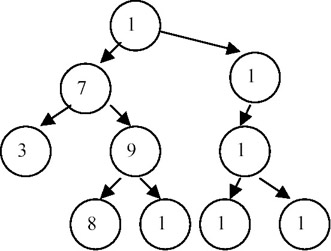The preorder traversal is 12, 7, 3, 9, 8, 11, 18, 14, 13, 15.

The stepwise run of the algorithm is as follows.

step

node

stack

output

0

nil

empty

nil

1

12

18 7

12

2

7

18 9 3

12 7

3

3

18 9

12 7 3

4

9

18 11 8

12 7 3 9

5

8

18 11

12 7 3 9

6

11

18

12 7 3 9 8 11

7

18

14

12 7 3 9 8 11 18

8

14

15 13

12 7 3 9 8 11 18 14

9

13

15

12 7 3 9 8 11 18 14 13

10

15

empty

12 7 3 9 8 11 18 14 13 15

Points to Remember

1. The preorder traversal needs a stack.
2. Keeping stack processing and tree processing separate helps us make the program modular. This can help us in using the same implementation of stack/queue for some other application, by making changes in only the data type contained in the stack/queue.
3. In the preorder traversal, the right child should be pushed in the stack first, followed by the left child.
4. The recursive version is more readable than the iterative version. This is because the tree data structure itself is inherently recursive.

## PROBLEM WRITE A NON RECURSIVE VERSION OF POSTORDER

Program

```/******************** stack.c *********************/
#include

#define SUCCESS 0
#define ERROR -1

struct tnode;

typedef struct tnode *stype;
typedef struct snode snode;
typedef struct snode *stack;

struct snode {
stype data;
snode *next;
};

void sInit( stack *s ) {
*s = NULL;
}

int sPush( stack *s, stype data ) {
/*
* push data in stack s.
*/
snode *ptr = (snode *)malloc( sizeof(snode) );
ptr->data = data;
ptr->next = *s;
*s = ptr;

return SUCCESS;
}

stype sPop( stack *s ) {
/*
* returns data at top of stack s.
*/
stype data;
snode *ptr;

if( sEmpty(*s) ) {
fprintf( stderr, "ERROR : popping from empty stack.
" );
return (stype)NULL;
}
data = (*s)->data;
ptr = *s;
(*s) = (*s)->next;
free(ptr);
return data;
}

int sEmpty( stack s ) {
return ( s == NULL );
}

/******************** main.c *********************/
#include
#include
#include "stack.c"

typedef int ttype;
typedef struct tnode tnode;

struct tnode {
ttype data;
tnode *left;
tnode *right;
};

tnode *tree = NULL;

int tInsert( ttype data ) {
/*
* insert data into global tree.
*/
tnode *ptr = (tnode *)malloc( sizeof(tnode) );
ptr->data = data;
ptr->left = NULL;
ptr->right = NULL;
tInsertPtr( &tree, ptr );
}

int tInsertPtr( tnode **tree, tnode *ptr ) {
/*
* inserts ptr into tree recursively.
*/
if( *tree != NULL ) {
if( ptr->data < (*tree)->data )
tInsertPtr( &((*tree)->left), ptr );
else
tInsertPtr( &((*tree)->right), ptr );
}
else
*tree = ptr;
return SUCCESS;
}

void tPrint( tnode *tree ) {
/*
* prints tree in inorder recursively.
*/
if( tree != NULL ) {
printf( "going left of %d...
", tree->data );
tPrint( tree->left );
printf( "%d ", tree->data );
printf( "going right of %d...
", tree->data );
tPrint( tree->right );
printf( "tree of %d is over.
", tree->data );
}
}

void tIterPostorder( tnode *tree ) {
/*
* prints tree in postorder iteratively using 2 stacks.
*/
stack s1, s2;
sInit(&s1); sInit(&s2);

if( tree != NULL ) {
sPush( &s1, tree );

while( !sEmpty(s1) ) {
tnode *t = sPop(&s1);
sPush( &s2, t );
if( t->left != NULL )
sPush( &s1, t->left );
if( t->right != NULL )
sPush( &s1, t->right );
}
while( !sEmpty(s2) )
printf( "%d
", sPop(&s2)->data );
}
}

int main() {
tInsert(4);
tInsert(2);
tInsert(5);
tInsert(1);
tInsert(3);
tInsert(6);
//tInsert(7);
tPrint(tree);
tIterPostorder(tree);
}
```

Explanation

1. In a postorder traversal, a node's left subtree is first output, followed by the right subtree, and finally the node is output. Thus, we need a stack in which we push the right child, followed by the left child. But we also need the node itself in the output. So, we will have to push it again in the stack before pushing its children. But then how will we keep track of whether that node is already processed? If we don't, we will again push it and its children in the stack, forming an infinite loop!
2. One way is to tag it as ‘processed.’ This can be done by adding a field to each node that will specify its status. Another way is to keep a list of processed nodes. We use this latter approach, maintaining a stack of processed nodes. The reason for using a stack is that when all the nodes in the tree are processed, the nodes in the stack give postorder traversal in reverse. Thus, by popping the nodes and outputting one by one, we get the required postorder traversal.
3. Example:The postorder traversal of this tree is 3, 8, 11, 9, 7, 13, 15, 14, 18, 12. The stepwise run of the algorithm is shown next:

step

node

stack 1

stack 2

0

nil

empty

empty

1

12

7 18

12

2

18

7 14

12 18

3

14

7 13

15 12 18 14

4

15

7 13

12 18 14 15

5

13

7 12

18 14 15 13

6

7

3 9

12 18 14 15 13 7

7

9

3 8 11

12 18 14 15 13 7 9

8

11

3 8

12 18 14 15 13 7 9 11

9

8

3

12 18 14 15 13 7 9 11 8

10

3

empty

12 18 14 15 13 7 9 11 8 3

Stack 2 can now be output to get the required postorder traversal of the tree.

Points to Remember

1. Iterative postorder requires a stack.
2. Keeping stack processing and tree processing separate helps us to make the program modular. This can help us in using the same implementation of the stack for some other application, by making changes in only the data type contained in the stack.
3. The left child of a node is first pushed to the stack, followed by the right child.
4. The recursive version is more readable than the iterative version. This is because the tree data structure itself is inherently recursive.

## PROBLEM PREORDER TRAVERSAL OF A THREADED BINARY TREE

Write a function to traverse an inorder threaded binary tree in preorder.

Program

```#include

#define SUCCESS 0
#define ERROR -1

typedef int type;
typedef struct node node;
typedef enum {FALSE, TRUE} bool;

struct node {
type data;
node *lchild, *rchild;
};

/* NOTE: since this is a threaded binary tree, there wont be any condition */
/* of type (ptr == NULL). */

node tree;

void tInit() {
tree.lchild = tree.rchild = &tree;
tree.data = 99999999;
}

node *insucc( node *t ) {
/*
* find inorder successor of t.
*/
node *temp = t->rchild;
temp = temp->lchild;
return temp;
}

node *inpred( node *t ) {
/*
* find inorder predecessor of t.
*/
node *temp = t->lchild;
temp = temp->rchild;
return temp;
}

int tInsertRight( node *s, node *t ) {
/*
* insert t as right child of s.
*/
node *temp;

t->rchild = s->rchild;
t->lchild = s;
s->rchild = t;
if( t->rthread == FALSE ) {
temp = insucc(t);
temp->lchild = t;

}
return SUCCESS;
}

int tInsertLeft( node *s, node *t ) {
/*
* insert t as left child of s.
*/
node *temp;

t->lchild = s->lchild;
t->rchild = s;
s->lchild = t;

if( t->lthread == FALSE ) {
temp = inpred(t);
temp->rchild = t;
}
return SUCCESS;
}

node *tGetNewNode( type data ) {
/*
* returns a new node containing the data.
*/
node *ptr = (node *)malloc( sizeof(node) );
ptr->data = data;
ptr->lchild = ptr->rchild = NULL;
return ptr;
}

int tInsert( node *t, type data ) {
/*
* insert data in t recursively.
*/
if( data < t->data )
tInsertLeft( t, tGetNewNode(data) );
else
tInsert( t->lchild, data );
else
tInsertRight( t, tGetNewNode(data) );
else
tInsert( t->rchild, data );
return SUCCESS;
}

void tPrint( node *t ) {
/*
* prints t inorder recursively without using threads.
*/
if( t != &tree ) {
tPrint( t->lchild );
printf( "%d
", t->data );
tPrint( t->rchild );
}
}

void tPrintPreorder( node *t ) {
/*
* prints tree preorder (no use of threads).
*/
if( t != &tree ) {
printf( "%d
", t->data );
tPrintPreorder( t->lchild );
tPrintPreorder( t->rchild );
}
}

void tPrintInorder( node *tree ) {
/*
* prints tree inorder using threads.
*/
node *temp = tree;
do {
temp = insucc(temp);
if( temp != tree )
printf( "%d
", temp->data );
} while( temp != tree );
}

int main() {
tInit();
tInsert( &tree, 4 );
tInsert( &tree, 2 );
tInsert( &tree, 1 );
tInsert( &tree, 3 );
tInsert( &tree, 6 );
tInsert( &tree, 5 );
tInsert( &tree, 7 );
tPrint( tree.lchild );
printf( "
" );
tPrintPreorder( tree.lchild );

return 0;
}
```

Explanation

1. In a threaded binary tree, the NULL links of leaf nodes are replaced by pointers (called threads) to other nodes in the tree. If prightchild is normally equal to NULL, it is replaced by a pointer to the node which would be printed after p when traversing the tree in inorder. A NULL leftchild link at node p is replaced by a pointer to the node that immediately precedes node p in inorder. The left link of the first node and the right link of the last node printed in the inorder traversal point to a dummy head node of the tree, and all the nodes appear in the left subtree of this head node. For example, in this representation, a tree such as the one shown next, where solid pointers are normal links and the dotted pointers are threads, t means TRUE and f means FALSE.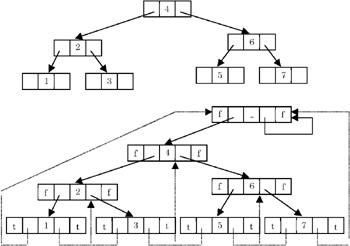2. In the memory representation, we should be able to distinguish between threads and normal pointers. This is done by adding two Boolean fields to the structure: leftthread and rightthread. If treeleftthread==TRUE, then treeleftchild contains a thread; otherwise, it contains a pointer to the leftchild. Similarly, if treerightthread == TRUE, then treerightchild contains a thread. Otherwise it contains a pointer to the rightchild.
3. The function to traverse a threaded binary tree remains as simple as that for the normal binary tree. One simply needs to check for a link not that is not a thread, and traverse it. So the recursive function tPrintPreorder() is self-explanatory. For example, the preorder traversal of the tree above is 4, 2, 1, 3, 6, 5, 7.

Points to Remember

1. Some traversing algorithms are simplified by making a tree threaded.
2. Making a tree threaded makes insertions and deletions clumsy. Also, the node size increases. So this increased complexity should be taken into consideration when using a threaded binary tree for an application.
3. Keeping a dummy head node helps in easy insertions, deletions, and traversals.

## PROBLEM IMPLEMENTATION OF A SET USING A BINARY TREE

Implement union() and find() operations on sets represented as trees.

Program

#include #define N 100 // max no of elements together in all sets. typedef int type; typedef struct node node; struct node { type val; // this is value of member. int parent; // this is index of parent in the array. }; node sets[N]; // all sets are contained in it. int setsindex = 0; // total no of elements in sets. int insertRoot( type val ) { /* * insert val in sets as a root of a new tree. */ sets[setsindex].val = val; sets[setsindex].parent = -1; setsindex++; return setsindex-1; } void insertElement( int rootindex, type val ) { /* * insert element val in set whose root is indexed at rootindex. */ sets[setsindex].val = val; sets[setsindex].parent = rootindex; setsindex++; } int buildSet( type a[], int n ) { /* * repeated calls to this fun with diff arrays will insert diff set in sets. * forms a tree representation of elements in a. * n is number of elements in the set. * empty set(n==0) cannot be represented here. * returns index of root. */ int i, rootindex; if( n <= 0 ) { fprintf( stderr, "n should be > 0. " ); return -1; } // check whether there is enough space for n elements. if( setsindex+n > N ) { fprintf( stderr, "ERROR: set overflow. " ); return -1; } // a becomes the root. rootindex = insertRoot( a ); for( i=1; i

Explanation

1.
2. We represent sets as trees in which different elements are stored as nodes in a tree. Since the order of elements is immaterial in sets, the order of nodes also does not matter in the tree. However, the links of the tree are reversed, that is, the child nodes have links to the parent instead of the parent pointing to its children. The reason for this representation is discussed next. Example: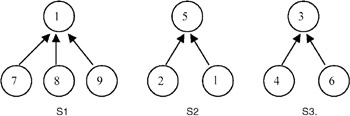3.
4. Union of two disjointed sets S1 and S2 (unionSets()) under the tree representation can be carried out simply by making any node of S1 the parent node of the root node of S2, or vice-versa. This operation can be carried out in a constant amount of time. Example:5.
6. The find operation (findSet()) searches for the root of an element in the tree. It travels from that element upwards in the hierarchy until it reaches a node that has no parent. Thus, its root is determined. The complexity of the find() operation is O(h) where h is the height of the tree. If the height remains below O(log n), the operation is faster. However, the worst-case time is linear. This O(n) time appears when each node (except the root) has only one predecessor. Example: ple: The root of vertex 8 in S1 in the last example is 1. However, the root of 8 in (S1 U S2) is 1 or 5 depending on how the union is done.
7.
8. The program stores all the sets in a global array of nodes. Each node contains the value of the element and the index of its parent element. To make find() efficient, each node is connected directly to the root node. The function unionSets(i1, i2) take indices of root elements and makes i1 the parent of the root node pointed to by i2. The function findSets(index) travels backwards from the node pointed to by the index until it reaches any of the root nodes. The root node has a parent index equal to –1.
9.

Points to Remember

1.
2. The function union() does not work if the sets are not disjointed.
3.
4. Operations union() and find() were devised from specific applications that use symbol tables. This asks for a specific representation of data in the form of a tree with reversed links. This shows how closely algorithms and data structures are related.
5.
6. union() has a complexily of O(1), while find() has the worst-case complexity of O(h), where h is the height of the element in the tree.
7.

## PROBLEM HUFFMAN CODING

Write a program to find Huffman codes for a set of characters, given the frequency of occurrence of each of these characters.

Program

```/********************** huffman.q.h ************************/
#include

typedef struct qnode qnode;
typedef tree qtype;
typedef struct {
qnode *front;
qnode *rear;
} queue;

struct qnode {
qtype op;
qnode *next;
};

bool qEmpty( queue *q ) {
return (q->front == NULL);
}

void qPushBack( queue *q, qtype op ) {
/*
* pushes op at q.rear.
*/
qnode *ptr = (qnode *)malloc( sizeof(qnode) );
ptr->op = op;
ptr->next = NULL;
if( qEmpty(q) ) // first element in q.
q->front = ptr;
else
q->rear->next = ptr;
q->rear = ptr;
}

void qInsertSorted(queue *q, qtype op) {
/*
* inserts val in sorted q and keeps new q sorted.
*/
qnode *ptr = (qnode *)malloc(sizeof(qnode));
qnode *curr, *prev;
ptr->op = op;

for(prev=NULL, curr=q->front; curr && curr->op->w < op->w; prev=curr, curr=curr->next)
;
if(!curr && !prev) // q empty.
ptr->next = NULL, q->rear = q->front = ptr;
else if(!curr) // op is the max value.
ptr->next = NULL, prev->next = q->rear = ptr;
else if(!prev) // op is the min value.
ptr->next = curr, q->front = ptr;
else { // if prev and ptr both exist.
ptr->next = curr;
prev->next = ptr;
}
}

qtype qPopFront( queue *q ) {
/*
* pops op from q->front.
*/
qnode *ptr = q->front;
qtype op;

if( qEmpty(q) )
return (qtype)NULL;
q->front = q->front->next;
if( qEmpty(q) ) q->rear = NULL;

op = ptr->op;
free(ptr);
return op;
}

/********************* huffman.c *************************/
#include

#define MAXLEN 80

typedef struct node node;
typedef char type;
typedef node *tree;
typedef enum {FALSE, TRUE} bool;

struct node {
int w;
type val;
node *left, *right;
};

#include "huffman.q.h"

int compare(const void *e1, const void *e2) {
/*
* compare the two elements in e1 and e2.
* each element is a vector of two elements.
*/
return ((int *)e1) > ((int *)e2);
}

void printTree(tree t, char *outputstr) {
/*
* print the huffman codes for each element of t.
* outputstr contains huffman code for t (NOT parent of t).
* assumes t!=NULL.
*/
char str = "1";

if(t->right) {
strcat(outputstr, str);
printTree(t->right, outputstr);
outputstr[strlen(outputstr)-1] = 0; // restore.
}
if(t->left) {
str = '0';
strcat(outputstr, str);
printTree(t->left, outputstr);
outputstr[strlen(outputstr)-1] = 0; // restore.
}
else if(!t->right)
printf("%c=%d=%s.
", t->val, t->w, outputstr);
}

tree buildTree(int a[], int n) {
/*
* build a huffman tree using frequency in a[i] where a[j] indicates
* the character
* for that sort a on frequency.
* n is the size of a.
*/
int i;
tree t = NULL;
queue sortedq = {NULL};

// sort a on frequency.
qsort(a, n, sizeof(a), compare);

// insert each element in tree.
for(i=0; iw = a[i];
temp->val = (type)a[i];
qPushBack(&sortedq, temp);
}
// assume n>0.
while(TRUE) {
tree t2 = NULL,
t1 = qPopFront(&sortedq);
if(!qEmpty(&sortedq))
t2 = qPopFront(&sortedq);
else {
t = t1;
break;
}

t = (tree)malloc(sizeof(node));
t->w = t1->w + t2->w;
t->left = t1;
t->right = t2;
{
qnode *ptr;
for(ptr=sortedq.front; ptr; ptr=ptr->next)
printf("%d ", ptr->op->w);
printf("
");
}
printf("insertsorted=%d.
", t->w);
qInsertSorted(&sortedq, t);
}
return t;
}

int main() {
int a[] = { {'a', 20},
{'b', 23},
{'c', 14},
{'d', 56},
{'e', 35},
{'f', 29},
{'g', 5 }
};
char outputstr[MAXLEN] = "";
tree t = buildTree(a, sizeof(a)/2/sizeof(int));

if(t)
printTree(t, outputstr);

return 0;
}
```

Explanation

1. Many applications prefer that frequently occurring messages have smaller lengths during coding, to make efficient use of the available bandwidth. This can be done by finding a binary tree with minimum weighted external path length. An external path length of a binary tree is the sum of all external nodes of the lengths of the paths, from the root to those nodes. The null nodes in a tree are called external nodes. Weighted external path length can be obtained by multiplying the external path length of each node by the frequency contained in the node, and than adding all of these values.
Example: ple: External path lengths of nodes A, B, C, D are 2, 2, 2, 2 and the weighted external path length of the tree is
2*2 + 2*4 + 2*5 + 2*15 = 52.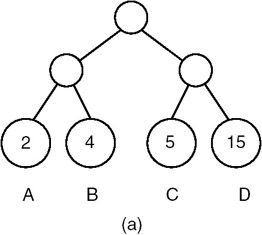Note that this is not the minimum weighted path length. The minimum weighted path length can be obtained by restructuring the tree as shown here.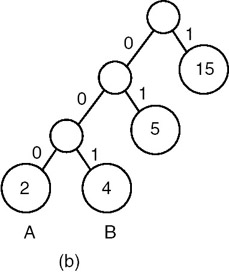The path length of this tree is 1*15 + 2*5 + 3*2 + 3*4 = 43, which is minimum. The numbers 0 and 1 of the edges will be clear in the following description.

2. An easy and nice solution to the problem of finding a binary tree with minimum weighted external path length is given by D. Huffman. We implement the algorithm in the function buildTree(). This algorithm first prepares a list of nodes out of the input characters and their frequencies. This list is sorted on the frequency in ascending order. Thus every retrieval retrieves the node with minimum frequency count from the list. The function then contains a loop that runs until the list becomes empty. In each iteration, two nodes (with minimum frequencies f1 and f2) are retrieved from the list, and another node with the frequency count f1+f2 is added to the list. This node also becomes the parent node of the two retrieved nodes. Thus every iteration reduces the length of the list by 1. In the end, when the list contains only one node, the node is returned, as the root of the Huffman tree is built.
3. If we assume that the codes contain only two symbols, 0 and 1, then the edge to a left child can be named 0 while that to a right child can be named 1, or vice versa, as given in (b) in the last example. Thus, the codes for A, B, C, and D are 000, 001, 01, and 1.

Points to Remember

1. Huffman codes are useful to encode a message using minimum number of symbols.
2. Another big advantage of Huffman codes is that given a message string built from Huffman codes, we can uniquely divide the message into the patterns of individual characters. For example, the pattern 01001101 uniquely identifies the character sequence CBDC.
3. The complexity of buildTree() is O(n logn+n+n^2) for qsort(), for loop, and while loop. Note that the complexity of qPushBack() is O(1) while that of qInsertSorted() is O(n). Thus the overall complexity of buildTree() is O(n^2). The complexity of qInsertSorted() may be improved by using a heap instead of a sorted list.

## PROBLEM IMPLEMENTATION OF A B TREE

Write procedures for insertion and deletion in a B-tree.

Program

```/************************* b.q.c *****************************/
#include
#include

typedef struct qnode qnode;
typedef btree qtype;

typedef struct {
qnode *front;
qnode *rear;
} queue;
struct qnode {
qtype op;
qnode *next;
};

bool qEmpty( queue *q ) {
return (q->front == NULL);
}

void qPush( queue *q, qtype op ) {
/*
* pushes op at q.rear.
*/
qnode *ptr = (qnode *)malloc( sizeof(qnode) );
ptr->op = op;
ptr->next = NULL;
if( qEmpty(q) ) // first element in q.
q->front = ptr;
else
q->rear->next = ptr;
q->rear = ptr;
}

qtype qPop( queue *q ) {
/*
* pops op from q->front.
*/
qnode *ptr = q->front;
qtype op;

if( qEmpty(q) )
return (qtype)-1;
q->front = q->front->next;
if( qEmpty(q) )
q->rear = NULL;

op = ptr->op;

free(ptr);

return op;
}

/*************************** b.c ****************************/
#include

#define K 5

typedef struct node node;
typedef int type;
typedef node *btree;
typedef enum {FALSE, TRUE} bool;
#include "b.q.c"

struct node {
type val[K]; // data in the node.
// actually first K-1 vals are valid. Kth entry is used
// during breaking of the node.
btree ptr[K+1]; // pointers to other nodes in the node.
// one extra ptr to be used in some loops.
int nptr; // no of pointers in the node = non-null ptrs.
// this can also be used to see whether a node is a leaf.
int nval; // no of values in the node.
// this is reqd even when nptr is present: for leaf nodes.
// for non-leaf nodes: nptr = nval+1.
// for leaf nodes: nptr = 0.
};

btree bNew() {
/*
* returns a new initialized node.
*/
btree tree = (btree)malloc(sizeof(node));
tree->nval = tree->nptr = 0;
return tree;
}

void insertVal(btree tree, type val, btree ptr) {
/*
* insert (val, ptr) in node pointed to by tree without any checks.
*/

int i, j;

for(i=0; inval && tree->val[i]val[i].
// shift-next the next values by one position.
for(j=tree->nval-1; j>=i; -j) {
tree->val[j+1] = tree->val[j];
tree->ptr[j+2] = tree->ptr[j+1];
}
// insert val now at i.
tree->val[i] = val;
tree->nval++;
tree->ptr[i+1] = ptr;
if(ptr != NULL) // tree is NOT a leaf.
tree->nptr++;
printf("	val %d inserted at position %d, tree->nptr=%d tree- >nval=%d.
", val, i, tree->nptr, tree->nval);
}

btree getSplitNode(btree tree) {
/*
* returns a new node containing vals and pointers from tree.
*/
int i, j;
btree ptr = bNew();

// copy vals.
for(i=(K-1)/2+1, j=0; ival[j] = tree->val[i];
ptr->nval = K/2;
tree->nval = K-K/2-1; // temporarily this node contains an extra val.

// copy ptrs.
for(i=(K-1)/2+1, j=0; i<=K; ++i, ++j)
ptr->ptr[j] = tree->ptr[i];
if(tree->nptr > 0) { // non-leaf nodes.
ptr->nptr = K/2+1;
tree->nptr = K-K/2;
}
else // leaf nodes.
tree->nptr = ptr->nptr = 0;
return ptr;
}

btree bMakeChanges(btree tree, btree ptr, btree parent) {
/*
* last val of tree contains an extra val which should be
* inserted in parent.
* if parent == NULL, then tree was root.
* tree = parent->ptr[i] if parent is NOT NULL.
*/
// extract the last value from tree.
type val = tree->val[tree->nval];
printf("in bMakeChanges().
");

if(parent == NULL) {
parent = bNew();
parent->ptr = tree;
parent->nptr = 1;
}
if(parent->nval < K-1) { // parent has space.
insertVal(parent, val, ptr);
}
else { // parent full.
printf("parent is full.
");
insertVal(parent, val, ptr);
return getSplitNode(parent);
}
return parent;
}

btree bInsert(btree tree, type val) {
/*
* calls insert to insert val in tree.
* if the return node is diff from tree, that means a new node has
* been created. Thus calls bMakeChanges().
*/
btree insert(btree tree, type val);

btree ptr = insert(tree, val);
if(ptr != tree) // node was split.
return bMakeChanges(tree, ptr, NULL);
return tree;
}

btree insert(btree tree, type val) {
/*
* inserts val in tree.
* returns tree if there is no change.
* if there is creation of a new node then the new node is returned.
*/
int i;
btree ptr;

if(tree->nptr > 0) { // non-leaf.
for(i=0; inval && tree->val[i]ptr[i].
printf("	val should be inserted in tree->ptr[%d].
", i);
ptr = insert(tree->ptr[i], val);
if(ptr != tree->ptr[i])
return bMakeChanges(tree->ptr[i], ptr, tree);
return tree;
}
else { // tree is a leaf.
if(tree->nval < K-1) { // space is available in the leaf.
insertVal(tree, val, NULL);
return tree;
}
else { // leaf full!
printf("	leaf is full.
");
insertVal(tree, val, NULL);
// now break the leaf node.
return getSplitNode(tree);
}
}
}

btree getAdjTree(btree tree, int offset, btree parent, int treeindex) {
/*
* returns parent[treeindex+offset] if exists else NULL.
*/
int newindex = treeindex+offset;
if(newindex >= 0 && newindex < parent->nptr)
return parent->ptr[newindex];
return NULL;
}

void combineNodes(btree left, btree right) {
/*
* left += right.
*/
int i;
int nptrleft = left->nptr;

for(i=0; inval; ++i)
left->val[left->nval++] = right->val[i];

for(i=0; inptr; ++i)
left->ptr[nptrleft+i] = right->ptr[i];
if(left->nptr > 0) // non-leaf.
left->nptr += i;
}

type getNextVal(btree ptr) {
/*
* return the first value in the first leaf accessible from ptr.
*/
if(ptr->nptr > 0) // still this is a non-leaf.
return getNextVal(ptr->ptr);
return ptr->val; // got it!
}

btree deleteVal(btree tree, int i) {
/*
* remove (val, ptr) at position i from tree without any checks.
* and return tree->ptr[i+1].
*/
btree rightptr = tree->ptr[i+1]; // ptr being removed along with val.

if(i == -1) // special case for a dummy call to this function.
return;

for(++i; inval; ++i) {
tree->val[i-1] = tree->val[i];
tree->ptr[i] = tree->ptr[i+1];
}
tree->nval-;

if(tree->nptr > 0) // if it is a non-leaf.
tree->nptr-;

return rightptr;
}

btree bApplyChanges(btree tree, btree parent, int treeindex) {
/*
* apply changes: tree is a non-leaf and tree = parent- >ptr[treeindex].
* also tree->nval < K/2.
*/
int parentvalindex;
btree returntree;
int offset = -1; // predecessor.

if(parent && tree->nval >= K/2)
return tree;
else if(parent == NULL && tree->nval == 0) {
free(tree);
return tree->ptr;
}
else if(parent == NULL)
return tree;

// parent is NOT NULL.

offset = 1; // successor.

extra val here too.
btree parentparent;
int parentindex;
type parentval;
btree returntree;

//printf("combine tree, parent median val, adjtree.
");
//printf("also check parent for having val[parentvalindex];
deleteVal(parent, parentvalindex);
// the return val is tree/adjtree. Hence dont worry.
if(offset == -1) {
// combine adjtree, parentval and tree.
free(tree);
}
else { // offset == 1: right sibling.
// combine tree, parentval and adjtree.
tree->val[tree->nval++] = parentval;
returntree = tree;
}
return returntree;
}
else {
}

}
else {
returntree = tree;
}
// adjtree has an extra val.
parentvalindex = (2*treeindex+offset)/2;
// insert parent val in tree.
insertVal(tree, parent->val[parentvalindex], returntree->ptr);
// now promote val in adjtree to parent.

return tree;
}

btree delete(btree tree, type val, int i, btree parent, int treeindex)
{
/*
* delete val from tree. val == tree->val[i].
* and tree == parent->nptr[treeindex].
*/
int parentvalindex;
btree bDelete(btree tree, type val, btree parent, int treeindex);

if(tree->nptr == 0) { // leaf.
deleteVal(tree, i);

// find two adjacent nodes to tree(succ and pred) and
// see whether any of them has >K/2 vals. if yes then bring
// the parent value between tree and the node into tree and
// promote the value in the adjacent node into the parent.
// if no such adjacent node exists, combine tree, parent val and
// if this leaves parent node having ptr[i+1]); // since tree->val[i] exists

// tree->val[i+1] exists.
tree->val[i] = nextval;
bDelete(tree->ptr[i+1], nextval, tree, i+1);
}
return bApplyChanges(tree, parent, treeindex);
}

btree bDelete(btree tree, type val, btree parent, int treeindex) {
/*
* delete val from tree if exists.
* tree == parent[treeindex].
*/
int i;

for(i=0; inval && tree->val[i]val[i] == val) {
printf("val=%d found: to be deleted.
", val);
return delete(tree, val, i, parent, treeindex);
}
else if(tree->nptr > 0) { // the val should be in a tree pointed to by tree->ptr[i].
//printf("val=%d may be in tree->ptr[%d].
", val, i);
bDelete(tree->ptr[i], val, tree, i);
//printf("now check tree for <50% values.
");
return bApplyChanges(tree, parent, treeindex);
}
else { // leaf reached.
printf("val=%d does NOT exist.
", val);
return tree;
}
}
void bPrintFormatted(btree tree) {
btree ptr;
int i;
queue q = {NULL};
qPush(&q, tree);
qPush(&q, NULL);

printf("---------------------------------------------------------------------------
");

while(qEmpty(&q) == FALSE) {
ptr = qPop(&q);
if(ptr) {
for(i=0; inval-1; ++i)
printf("%d-", ptr->val[i]);
if(inval)
printf("%d ", ptr->val[i]);
for(i=0; inptr; ++i)
qPush(&q, ptr->ptr[i]);
}
else {
printf("
");
if(qEmpty(&q) == FALSE)
qPush(&q, NULL);
}
}
printf("---------------------------------------------------------------------------
");
}

int main() {
btree tree = bNew();
tree = bInsert(tree, 1);
tree = bInsert(tree, 2);
tree = bInsert(tree, 4);
tree = bInsert(tree, 3);
tree = bInsert(tree, 5);
tree = bInsert(tree, 6);
tree = bInsert(tree, 7);
tree = bInsert(tree, 8);
tree = bInsert(tree, 9);
tree = bInsert(tree, 10);
tree = bInsert(tree, 11);
tree = bInsert(tree, 12);
tree = bInsert(tree, 13);
tree = bInsert(tree, 14);
tree = bInsert(tree, 15);
tree = bInsert(tree, 16);
tree = bInsert(tree, 17);
bPrintFormatted(tree);
tree = bDelete(tree, 6, NULL, -320);
bPrintFormatted(tree);
tree = bDelete(tree, 9, NULL, -320);
bPrintFormatted(tree);

return 0;
}
```

Explanation

1. A B-tree of order K is a K-way search tree that is either empty or is of height > 0 and satisfies the following properties:

1. the root node has at least two children.
2. all nodes other than the root node and leaf nodes have at least ceil(K/2) children.
3. all leaf nodes are at the same level.

It is easier to insert and delete nodes into a B-tree retaining the B-tree properties than it is to maintain the best possible K-way search tree at all times. Thus, the reasons for using B-trees rather than optimal K-way search trees for indices are the same as those for using AVL trees, as opposed to optimal binary search trees, when maintaining dynamic internal tables.

2. Each B-tree node of order K has space for K pointers and K – 1 values. We maintain an extra space for one pointer and one value which will be useful in insertion. We maintain counts of the number of values and number of pointers present in the node. Thus the node structure for a B-tree is as follows:

```typedef struct node *btree;
struct node {
type val[K];
btree ptr[K+1];
int nptr;
int nval;
};
```

nptr is the number of non-null pointers and nval is the number of valid values in a node. For a leaf-node, nptr == 0, while for all other nodes, nptr == nval + 1.

3. Insertion in a B-tree takes place by traversing the search tree for the value starting from root. When the search terminates in a leaf node, the value is inserted in the node. The node is then checked to see if thus more than K – 1 values. If the node has less than K values, the insertion is done. Otherwise, the node is split into two nodes of sizes floor(K/2) and the middle value is propagated into the parent node. This procedure is repeated until either the insertion stops (as there is space for the new value in a node) or nodes at all the levels in the tree get split and, finally, a new root node needs to be created. The value propagated from the original root is then put into the new root node and its pointers are adjusted accordingly.

Examples: Orignal tree of order K = 3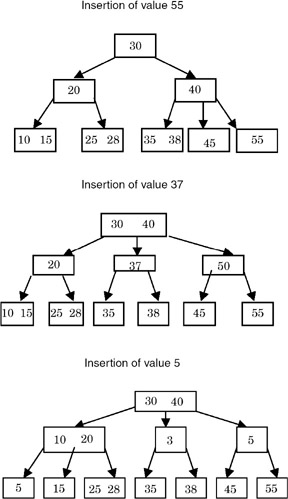4. Deletion of a value from a B-tree can be done as simply as performing an insertion. If, even after deletion of the value from a leaf node p, the node contains >= K/2 values, then nothing needs to be done. Otherwise the sibling nodes are checked to see if they have > K/2 values. If such a sibling q exists, then the value in the parent node between the two adjacent leaf nodes is demoted to node p, and a value in node q is promoted to the parent (this is called rotation). However, if the value v to be deleted is in a non-leaf node p, then the next value (w) to v in the search order (which appears in the right subtree of v in the leaf node) is promoted at the place of v and the value w is deleted from the leaf node. If, in this promotion and demotion, the parent node r contains less than K/2 values, then its adjacent node s is promoted to its parent t and the value in t is demoted to r. Also, the adjacent pointer to the value promoted from s becomes the adjacent pointer to the parent value that has been newly inserted in r.

Examples: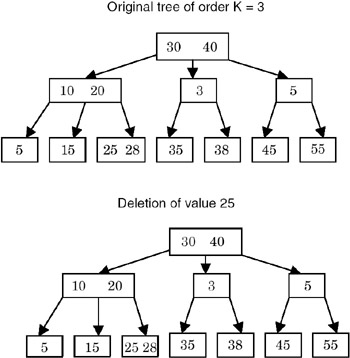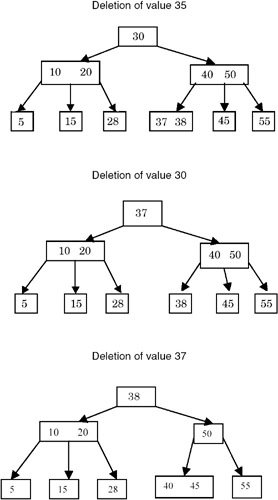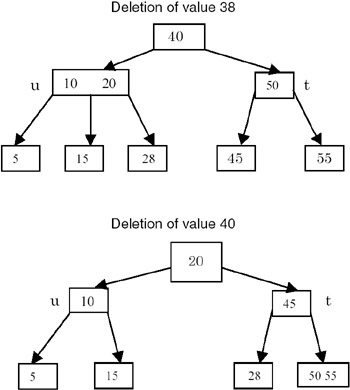Note that in the deletion of value 40, since it is a non-leaf node, its next value in the search order, 45, is promoted to its place so that 45 appears in the root. Then 45 is deleted from the leaf node r. After deletion, r has less than K/2 values. So its sibling s is checked for any extra values. s, with a value of 55, has only K/2 values. So the values of r, s, and 50 (the value of the parent node t between the two children) are combined to get a node with the values (50, 55). Now t contains less than K/2 values. Since it is a non-leaf node, it checks its adjacent node to see if it has more than K/2 values. The node u, with the values (10, 20), actually has more than K/2 values. So the last value of u, 20, is promoted to the root and the value 45 is demoted to t. The rightmost sibling of u (the node containing a value of 28) becomes the leftmost sibling of t. 20, the rightmost sibling of u, becomes the leftmost sibling of t. A symetric transformation is done when the adjacent node appears in the right. If none of the adjacent nodes of t contain any extra values, then t, u, and the value in their parent node between these two pointers are combined to form one node. Then their parent node is checked to see if it has less than K/2 values, and the procedure continues.

5. The function hierarchy for insertion and deletion appears the same. For insertion, the main driver function is bInsert(), which calls insert() and checks its return value to see whether the level was changed in the function. If it was, then bMakeChanges(), which propagates this change upwards in the tree is called. insertVal() is the function that puts a value (along with its right pointer) in a node. The function getSplitNode() splits a node in two parts, each containing K/2 values. The (K+1)th value in the node being split is stored in the first node as the (K/2)th value, which is then used in propagation. insert() is the main function that checks whether the node is a leaf or a non-leaf, and accordingly calls the different functions that have jsut been described.

For deletion, the main driver function is bDelete(), which calls itself recursively until it reaches a node that contains the value being deleted. It then calls delete(), which checks for the node to be a leaf or a non-leaf node and takes appropriate action as just described.

During insertion, bApplyChanges() is called from bDelete() and delete(). bApplyChanges is similar to bMakeChanges, and does the job of demotion and propagation of values in the current node and its siblings. It uses a function called getAdjTree(), which returns a node adjacent to a node with the same parent. combineNodes(), a procedure complementary to getSplitNode(), combines the second node with the first. In order to get the next value in the search order from the tree, the function getNextVal() is called. deleteVal() is a function that is complementary to the function insertVal(), and is used to delete a value, along with its right pointer, from a node. It then returns the pointer.

6. The procedure bPrintFormatted() uses a queue to print the tree in the breadth-first manner.

Points to Remember

1. In a B-tree of order K, there can be at most K pointers in a node and K – 1 values.
2. All the leaf nodes of a B-tree are at the same level.
3. The complexity of both insertion and deletion is O(h), where h is the height of the B-tree. This is because of the propagation of values upwards during insertion and downwards during deletion.
4. By writing the functions of insertion and deletion in a similar manner, the code becomes easier to write and understand.
5. A queue is required for printing a tree in the breadth-first format.
6. Searching for a value inside a node can be done using binary search as the values are sorted. However, if K is small enough, even the linear search will give a similar performance.

## PROBLEM IMPLEMENTATION OF A B+ TREE

Write procedures for insertion and deletion in a B+ tree.

Program

```/*********************** bplus.q.c **************************/
#include
#include

typedef struct qnode qnode;
typedef btree qtype;
typedef struct {
qnode *front;
qnode *rear;
} queue;

struct qnode {
qtype op;
qnode *next;
};
bool qEmpty( queue *q ) {
return (q->front == NULL);
}

void qPush( queue *q, qtype op ) {
/*
* pushes op at q.rear.
*/
qnode *ptr = (qnode *)malloc( sizeof(qnode) );
ptr->op = op;
ptr->next = NULL;
if( qEmpty(q) ) // first element in q.
q->front = ptr;
else
q->rear->next = ptr;
q->rear = ptr;
}

qtype qPop( queue *q ) {
/*
* pops op from q->front.
*/
qnode *ptr = q->front;
qtype op;

if( qEmpty(q) )
return (qtype)-1;
q->front = q->front->next;
if( qEmpty(q) )
q->rear = NULL;

op = ptr->op;
free(ptr);

return op;
}

/************************** bplus.c *************************/
#include
#include
#define K 5

typedef struct node node;
typedef int type;
typedef node *btree;
typedef enum {FALSE, TRUE} bool;

#include "bplusq.c"

struct node {
type val[K]; // data in the node.
// actually first K-1 vals are valid. Kth entry is used
// during breaking of the node.
btree ptr[K+1]; // pointers to other nodes in the node.
// one extra ptr to be used in some loops.
int nptr; // no of pointers in the node = non-null ptrs.
// this can also be used to see whether a node is a leaf.
int nval; // no of values in the node.
// this is reqd even when nptr is present: for leaf nodes.
// for non-leaf nodes: nptr = nval+1.
// for leaf nodes: nptr = 0.
node *right; // if this is a leaf node, this points to its sibling.
};

btree bNew() {
/*
* returns a new initialized node.
*/
btree tree = (btree)malloc(sizeof(node));
tree->nval = tree->nptr = 0;
tree->right = NULL;
return tree;
}

void insertVal(btree tree, type val, btree ptr) {
/*
* insert (val, ptr) in node pointed to by tree without any checks.
*/
int i, j;

for(i=0; inval && tree->val[i]val[i].
// shift-next the next values by one position.
for(j=tree->nval-1; j>=i; --j) {
tree->val[j+1] = tree->val[j];
tree->ptr[j+2] = tree->ptr[j+1];
}
// insert val now at i.
tree->val[i] = val;
tree->nval++;
tree->ptr[i+1] = ptr;
if(ptr != NULL) // tree is NOT a leaf.
tree->nptr++;
printf("	val %d inserted at position %d, tree->nptr=%d tree- >nval=%d.
", val, i, tree->nptr, tree->nval);
}

btree getSplitNode(btree tree) {
/*
* returns a new node containing vals and pointers from tree.
*/
int i, j;
btree ptr = bNew();

// copy vals.
for(i=(K-1)/2+1, j=0; ival[j] = tree->val[i];
ptr->nval = K/2;
if(tree->nptr > 0) // non-leaf node.
tree->nval = K-K/2-1; // temporarily this node contains an extra val.
else // leaf node.
tree->nval = K-K/2;

// copy ptrs.
//for(i=(K-1)/2, j=0; i<=K; ++i, ++j)
for(i=(K-1)/2+1, j=0; i<=K; ++i, ++j)
ptr->ptr[j] = tree->ptr[i];
if(tree->nptr > 0) { // non-leaf nodes.
ptr->nptr = K/2+1;
tree->nptr = K-K/2;
}
else { // leaf nodes.
tree->nptr = ptr->nptr = 0;
tree->right = ptr; // bplus tree: list of leaves.
}
return ptr;
}

btree bMakeChanges(btree tree, btree ptr, btree parent) {
/*
* last val of tree should be inserted in parent.
* if parent == NULL, then tree was root.
* tree = parent->ptr[i] if parent is NOT NULL.
*/
// extract the last value from tree.
type val = (tree->nptr>0 ? tree->val[tree->nval] : tree->val[tree- >nval-1]);
printf("in bMakeChanges().
");

if(parent == NULL) {
parent = bNew();
parent->ptr = tree;
parent->nptr = 1;
}
if(parent->nval < K-1) { // parent has space.
insertVal(parent, val, ptr);
}
else { // parent full.
printf("parent is full.
");
insertVal(parent, val, ptr);
return getSplitNode(parent);
}
return parent;
}

btree bInsert(btree tree, type val) {
/*
* calls insert to insert val in tree.
* if the return node is diff from tree, that means a new node has
* been created. This calls bMakeChanges().
*/
btree insert(btree tree, type val);

btree ptr = insert(tree, val);
if(ptr != tree) // node was split.
return bMakeChanges(tree, ptr, NULL);
return tree;
}

btree insert(btree tree, type val) {
/*
* inserts val in tree.
* returns tree if there is no change.
* if there is creation of a new node then the new node is returned.
*/
int i;
btree ptr;

if(tree->nptr > 0) { // non-leaf.
for(i=0; inval && tree->val[i]ptr[i].

printf("	val should be inserted in tree->ptr[%d].
", i);
ptr = insert(tree->ptr[i], val);
if(ptr != tree->ptr[i])
return bMakeChanges(tree->ptr[i], ptr, tree);
return tree;
}
else { // tree is a leaf.
if(tree->nval < K-1) { // space is available in the leaf.
insertVal(tree, val, NULL);
return tree;
}
else { // leaf full!
printf("	leaf is full.
");
insertVal(tree, val, NULL);
// now break the leaf node.
return getSplitNode(tree);
}
}
}
tree = bInsert(tree, 6);
tree = bInsert(tree, 7);
tree = bInsert(tree, 8);
tree = bInsert(tree, 9);
tree = bInsert(tree, 10);
tree = bInsert(tree, 11);
tree = bInsert(tree, 12);
tree = bInsert(tree, 13);
tree = bInsert(tree, 14);
tree = bInsert(tree, 15);
tree = bInsert(tree, 16);
tree = bInsert(tree, 17);
bPrintFormatted(tree);

tree = bDelete(tree, 6, NULL, -320);
bPrintFormatted(tree);
tree = bDelete(tree, 5, NULL, -320);
bPrintFormatted(tree);
tree = bDelete(tree, 7, NULL, -320);
bPrintFormatted(tree);
tree = bDelete(tree, 8, NULL, -320);
bPrintFormatted(tree);
tree = bDelete(tree, 9, NULL, -320);
bPrintFormatted(tree);
tree = bDelete(tree, 10, NULL, -320);
bPrintFormatted(tree);
tree = bDelete(tree, 11, NULL, -320);
bPrintFormatted(tree);

printf("The linked list of leaf nodes:
");
for(; ptr; ptr=ptr->right) {
for(i=0; inval; ++i)
printf("%d-", ptr->val[i]);
printf(" ");
}
printf("
");
return 0;
}
```

Explanation

1. A B+ tree of order K is a K-way search tree that is either empty or is of height > 0 and satisfies the following properties:

1. The root node has at least two children.
2. All nodes other than the root node and leaf nodes have at least ceil(K/2) children.
3. All leaf nodes are at the same level.
4. All values appear in the leaf nodes.
5. The leaf nodes are linked from left to right.
2. Each B+ tree node of order K has space for K pointers and K – 1 values. We maintain extra space for one pointer and one value, which will be useful in insertion. We maintain counts of the number of values and the number of pointers present in the node. For the linked list of leaf nodes, another pointer is needed. Thus the node structure for a B+ tree is as follows:

```typedef struct node *btree;
struct node {
type val[K];
btree ptr[K+1];
int nptr;
int nval;
node *right;
};
```

nptr is the number of non-null pointers and nval is the number of valid values in a node. For a leaf node, nptr == 0 while for all other nodes, nptr == nval + 1. The pointer right points to the node to the right of this node.

3. Insertion in a B+ tree takes place by traversing the search tree for the value starting from the root. The search always terminates in a leaf node. The value is inserted in the leaf node. The node is then checked to see if it has more than K–1 values. If the node has less than K values, the insertion is performed. Otherwise, the node is split into two nodes of sizes floor(K/2) and the middle value is ‘copied’ into the parent node. In case of a non-leaf node, the middle value is ‘moved’ to the parent node. This procedure is repeated until either the insertion stops (as there is space for the new value in a node) or nodes at all the levels in the tree get split, and finally a new root node needs to be created. The value propagated from the original root is then put into the new root node and its pointers are adjusted accordingly.

Examples: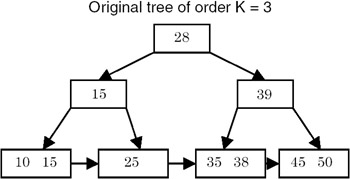Note that for a value v in a node and its two adjacent pointers on left and right, all values on left are £ v and all values on right are > v.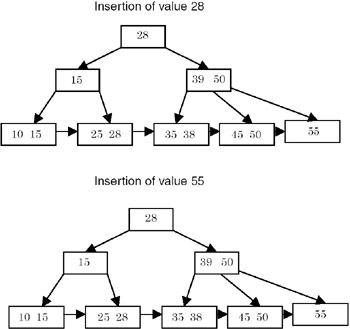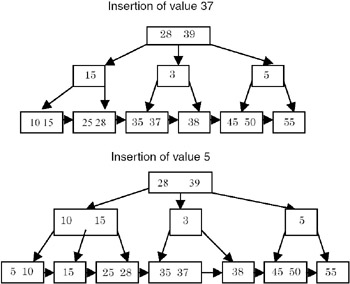4. Deletion of a value from a B+ tree can be done as simply as insertion is done. If, even after deletion of the value from a leaf node p, the node contains >= K/2 values, then nothing needs to be done. Otherwise the sibling nodes are checked for > K/2 values. If such a sibling q exists, then the adjacent value in q is moved to the node p and the value in the parent node between the two leaf nodes is replaced by the last value in its left child. If such a sibling q does not exist, then nodes p and q are combined, and the value in the parent node between these two leaf nodes is deleted. If in this process, the parent node r contains less than K/2 values, then its adjacent node s is promoted to its parent t and the value in t is demoted to r. Also, the adjacent pointer to the value promoted from s becomes the adjacent pointer to the parent value newly inserted in r.

Examples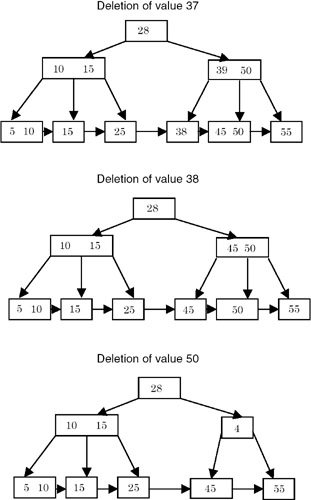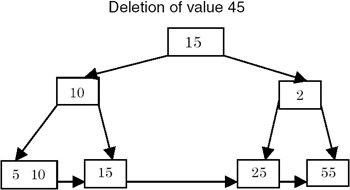Note that the value 45 is deleted from the leaf node r. After deletion, r has less than K/2 values. So its sibling s is checked for any extra values. s, with a value of 55, has only K/2 values. So the values of r, s, and 50 (the value of the parent node t between the two children), are combined to get a node with the value 55, and the value 45 is deleted from t, along with its right pointer. r's right pointer is appropriately updated. Now t contains less than K/2 values. Since it is a non-leaf node, it checks its adjacent node to see if it has more than K/2 values. The node u, with the values (10, 15) actually has more than K/2 values. So the last value of u, 15, is promoted to the root and the value 28 is demoted to t. The rightmost sibling of u (the node containing a value of 25) becomes the leftmost sibling of t. A symmetric transformation is done when the adjacent node appears in the right. If none of the adjacent nodes of t contain any extra values, then the values of r, u, and the value in their parent node between these two pointers are combined to form one node. Then their parent node is checked to see if it has less than K/2 values, and the procedure continues.

5. The function hierarchy for insertion and deletion appears the same. For insertion, the main driver function is bInsert(), which calls insert() and checks its return value to see whether the level was changed in the function. If it was, bMakeChanges() is called, which propagates this change upwards in the tree. insertVal() is the function that puts a value (along with its right pointer) in a node. The function getSplitNode() splits a node in two parts, each containing K/2 values. The (K+1)th value in the node being split is stored in the first node as the (K/2)th value, which is then used in propagation. insert() is the main function that checks whether the node is a leaf or a non-leaf and accordingly calls the different functions that have just been described.

For deletion, the main driver function is bDelete(), which calls itself recursively until it reaches the leaf node that contains the value being deleted. It then calls delete(), which takes appropriate action as just described. During insertion, bApplyChanges() is called from bDelete() and delete(). bApplyChanges is similar to bMakeChanges, and does the job of demotion and propagation of values in the current node and its siblings. It uses a function called getAdjTree(), which returns a node adjacent to a node with the same parent. combineNodes(), a procedure complementary to getSplitNode(), combines the second node with the first. In order to get the next value in the search order from the tree, the function getNextVal() is called. deleteval() is a function that is complementary to the function insertVal(), and is used to delete a value, along with its right pointer, from a node. It then returns the pointer.

6. The procedure bPrintFormatted() uses a queue to print the tree in the breadth-first manner.

Points to Remember

1. In a B+ tree of order K, there can be at most K pointers in a node and K–1 values.
2. All the leaf nodes of a B+ tree are at the same level.
3. All the inserted values in a B+ tree are present in leaf nodes. The non-leaf nodes may contain values that are not present in leaf nodes.
4. The complexity of both insertion and deletion is O(h), where h is the height of the B-tree. This is because of the propagation of values upwards during insertion and downwards during deletion.
5. A queue is required for printing a tree in the breadth-first format.
6. Searching for a value inside a node can be done using binary search as the values are sorted. However, if K is small enough, even the linear search will give a similar performance.
7. The updation of a value can be simulated by deletion followed by insertion.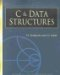C & Data Structures (Charles River Media Computer Engineering)
ISBN: 1584503386
EAN: 2147483647
Year: 2006
Pages: 232